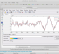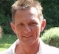## Proving Time Invariance of a systemStarted by 1 year ago6 replieslatest reply 1 year ago376 views
I did come across some useful posts here about how to go about proving time invariance of a system but I had this question.  Given something like this for instance y = x(t) + x(t-2), can we assume x(t) to be a rectangular pulse or some such signal and then prove time invariance using MATLAB?  Plot y and then shift it by 1 and then shift x by 1 and then plot result of shifted x input into y?  I am in a Signals and Systems class and we are following Oppenheim he uses abstract proofs for this sort of problem which I am unable to grasp.
[ - ]It will be worth your time to figure out which step in the proof loses your comprehension.  "Abstraction" just means a higher level of meaning - with lots of details hidden.  If you understand the details, then each level of abstraction makes understanding complex systems easier.  When you solve a problem, all the details are invoked - or the math won't work.  It's like writing a matrix equation instead of 4 equations separately.  The page shows a single symbol for the matrix, but Matlab has to hold every value of the matrix.

It's hard work to expand the details line by line.  Take your time and grind through it, if takes a couple of days then grind through it for a couple of days. After you do that for one really hard problem, the next one won't be quite as hard.  If there is a specific step that is clear you lose it, then you have some good questions to ask - don't be afraid to ask your professor, they want you to learn this.

You don't need to create proofs, but you should be able to follow them.  Figure out why you can't follow it, and ask specific questions until you can.  You'll be happy you did.

Patience, persistence, truth,

Dr. mike

[ - ]Hi,

For time invariance, you have to check whether if the shifted input signal also leads to the same output shifted by the same amount of time delay.

i.e.

y(t) = x(t) * t

lets give input x(t-3) -output is- -->  x(t-3) * t

but this is not equal to y(t-3) = x(t-3) * (t-3)

So this is not a time invariant system.

FOr your system, the system is time invariant.

x(t-t0) --> x(t-t0) + x(t-t0-2)

which equals y(t-t0)

You can find much more examples in the internet.

[ - ]Using Matlab, you cannot prove anything in the mathematic or physical realm.
Since Matlab is nothing but a tool.
Like a desktop calculator, which can help you for some calculation, it can help you to simplify a formula or find any good idea.

A proof, however, requires that you obey constraints and rules like exactitude, completeness and correctness.

As an example: If you use the desktop calculator to prove if (1/x)*x=1 you might end up with any possible result (because of rounding errors, and depending if you check the value 0).
Similarly, using Matlab, you might end up with a confirmation or a failure depending on how you do it. But whatever result you get, it's not the level of a proof.

Hence, both tools are recommendable tools during the investigation phase to get a feeling of the problem.

But for the proof: paper and pencil should do.

Bernhard

[ - ]I believe the OP was about using matlab as a learning tool.

For that Matlab and other tools are absolutely great compared to lectures or books or lengthy pages of equations.

I also believe such tools are used by many as a lead to end up with rules and equations which are then presented as if they never used the tool.

In the right hands processing millions and millions of data is no desktop calculator.

[ - ]Kirandan.

Hi. Here's a numerical example of your system y = x(t) + x(t-2):

n = 3:10;
X1 = [1,3,4,4,2,10,7,8,7,4] % Original input
Y1(n) = X1(n)-X1(n-2) % Original output

n = 3:11;
X2 = [0, X1] % Input X2 is X1 shifted forward (advanced) by one sample
Y2(n) = X2(n)-X2(n-2) % Output from "shifted" input X2

X1 = 1,3,4,4,2,10,7,8,7,4

Y1 = 0,0,3,1,-2,6,5,-2,0,-4

X2 = 0,1,3,4,4,2,10,7,8,7,4

Y2 = 0,0,3,3,1,-2,6,5,-2,0,-4

Notice how:

the 4th Y2 sample equals the 3rd Y1 sample,

the 5th Y2 sample equals the 4th Y1 sample,

the 6th Y2 sample equals the 5th Y1 sample,

the 7th Y2 sample equals the 6th Y1 sample,

etc.

[ - ]Understanding the mathematics is no doubt important.At the same time getting intution is equally important.Writing few lines of code in Matlab and plotting will give the intution.

Consider only one input x(t)=sin(2*pi*100*t);

plot x(t) for t=1:10000;

let y(t)=t*x(t);

plot y(t);

plot y(t-3);

plot t*x(t-3) --> This is the output for shifted input x(t) by 3.

plot y(t-3) and t*x(t-3) onthe same figure using hold and different colors.

They will not be the same.

Make sure when the index is negative or zero fill it with zeros.Else Matlab will give Error.

In your example if you take y(t)=x(t)+x(t-2) they will be same.Because it is time invariant.

Murali